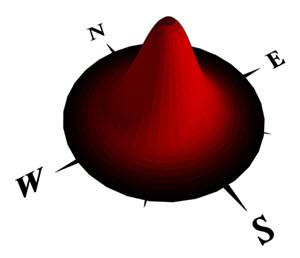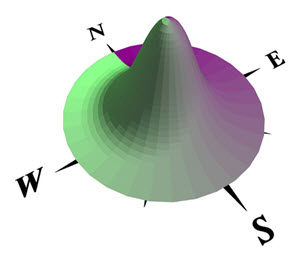# geometry function

## geometry.angle function

### Syntax

• float geometry.angle(angleSelector)

### Parameters

1. angleSelectorfloat
Selector for the angle calculation. Valid selectors are:
• maxSlope—calculates the maximum slope of the shape, relative to the xz-plane, in degrees.
• azimuth—calculates the azimuth of the direction of the current shape's maximum slope, in degrees;
• zenith—calculates the difference between 90 degrees (zenith) and the maximum slope.

### Returns

Angle of the current shape's geometry, depending on the selector, in degrees.

### Description

The geometry.angle function calculates the angle of current shape's geometry. azimuth is the horizontal angle measured clockwise from the north, i.e. 90 degrees is east, 180 degrees is south and 270 degrees is west. The azimuth is in the range [0, 360]. The geometry.angle(zenith) function returns the same value as ( 90 - geometry.angle(maxSlope) )

### Examples

 ``````Cone-->> comp(f) { all : color(geometry.angle(maxSlope) / 90, 0.0, 0.0) Shape. }````````````Cone-->> comp(f) { all : color(0.5, geometry.angle(azimuth) / 360, 0.5) Shape. }``````## geometry.area function

### Syntax

• float geometry.area()
• float geometry.area(areaSelector)

### Parameters

1. areaSelectorfloat
Selector for the faces to include in the area calculation. Valid selectors are:
• {surface| all } (default)
• { front | back | left | right | top | bottom }
• { object.front | object.back | object.left | object.right | object.top | object.bottom | object.side }
• { world.south | world.north | world.west | world.east | world.up | world.down | world.side }
• { street.front | street.back | street.left | street.right | street.side }

### Returns

Surface area of the current shape's geometry, depending on the provided area selector.

### Description

The geometry.area function calculates the surface area that is the sum of all its faces.

## geometry.boundaryLength

### Syntax

• float geometry.boundaryLength()

### Returns

The sum of all boundary edge lengths. An edge is considered a boundary edge if it belongs to no more than one face.

## geometry.{du|dv} functions

### Syntax

• float geometry.du(float uvSet, surfaceParameterization)
• float geometry.dv(float uvSet, surfaceParameterization)

### Parameters

1. uvSetinteger (range[0,9])
Number of texture coordinates set. The numbering corresponds to the texture layers of the material attribute, see also texturing: essential knowledge.
2. surfaceParameterizationselstr (range[0,1])

The surface parameter space: uvSpace or unitSpace. While uvSpace selects the actual texture coordinates, unitSpace calculates the geometry-dependent surface stretch along the u- or v- axis and calculates an approximation in world coordinate units (e.g. meters).

### Returns

The range (i.e. max - min) spanned by the u- or the v-coordinate, respectively, of the selected uvset.

## geometry.height function

### Syntax

• float geometry.height()

### Returns

The height of the geometry in world coordinates.

## geometry.isClosedSurface function

### Syntax

• bool geometry.isClosedSurface()

### Returns

trueif the geometry contains at least one face and all edges belong to exactly 2 faces, false otherwise.

## geometry.isConcave function

### Syntax

• bool geometry.isConcave()

### Returns

true if the geometry contains at least one concave face, false otherwise.

## geometry.isInstanced function

### Syntax

• bool geometry.isInstanced()

### Returns

True if the current shape's geometry is the instance of an asset, i.e. the geometry has not been altered since the insert operation , false otherwise.

### Description

The geometry.isInstanced() function can be used to e.g. check if an inserted asset was trimmed.

## geometry.isOriented function

### Syntax

• bool geometry.isOriented()
• bool geometry.area(areaSelector)

### Parameters

1. orientationSelectorbool
Selector for the faces to check for. Valid selectors are:
• { front | back | left | right | top | bottom | side }
• { object.front | object.back | object.left | object.right | object.top | object.bottom | object.side }
• { world.south | world.north | world.west | world.east | world.up | world.down | world.side }
• { street.front | street.back | street.left | street.right | street.side }

### Returns

true if at least one of the geometry's faces matches the orientationSelector, false otherwise.

### Description

The geometry.isOriented function can be used to determine a shape's orientation.

## geometry.isPlanar function

### Syntax

• bool geometry.isPlanar(tolerance)

### Parameters

1. tolerancefloat
The tolerance in degrees for deciding if an face is planar or not. The face normal is compared to the edges' cross product (''local normal'') at every vertex; if the angle between a ''local normal'' and the face normal is larger than the tolerance the face is non-planar. A reasonable value is 0.25 degrees.

### Returns

true if the geometry contains at least one face and all faces of are planar (within tolerance), false otherwise).

## geometry.isRectangular function

### Syntax

• bool geometry.isRectangular(float tolerance)

### Parameters

1. tolerancefloat
The tolerance in degrees for deciding if an angle is a right one or not.

### Returns

true if the geometry contains at least one face and all faces consist of 4 vertices and contain only right angles, false otherwise. Angles in the range [90-tolerance, 90+tolerance] are considered to be "right".

## geometry.nEdges function

### Syntax

• float geometry.nEdges()

### Returns

The (integral) number of edges of the current shape's geometry.

## geometry.nFaces function

### Syntax

• float geometry.nFaces()

### Returns

The (integral) number of faces of the current shape's geometry.

## geometry.nHoles function

### Syntax

• float geometry.nHoles()

### Returns

The (integral) number of holes (i.e. holes in faces) of the current shape's geometry.

## geometry.nVertices function

### Syntax

• float geometry.nVertices()

### Returns

The (integral) number of vertices of the current shape's geometry.

## geometry.{uMin|uMax|vMin|vMax} functions

### Syntax

• float geometry.uMin()
• float geometry.uMax()
• float geometry.vMin()
• float geometry.vMax()
• float geometry.uMin(float uvSet)
• float geometry.uMax(float uvSet)
• float geometry.vMin(float uvSet)
• float geometry.vMax(float uvSet)

### Parameters

1. uvSetinteger (range[0,9)
Number of texture coordinates set. The numbering corresponds to the texture layers of the material attribute, see also texturing: essential knowledge.

### Returns

The minimal or maximal u or v value of the selected uvset (the versions without parameter use uvset 0).

## geometry.volume function

### Syntax

• float geometry.volume()

### Returns

Volume of the current shape's geometry.